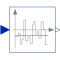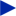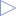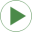# ContinuousMean

Calculates the empirical expectation (mean) value of its input signal# Information

This information is part of the Modelica Standard Library maintained by the Modelica Association.

This block continuously calculates the mean value of its input signal. It uses the function:

```    integral( u over time)
y = ----------------------
time - startTime```

This can be used to determine the empirical expectation value of a random signal, such as generated by the Noise blocks.

The parameter t_eps is used to guard against division by zero (the mean value computation starts at <simulation start time> + t_eps and before that time instant y = u).

This block is demonstrated in the examples UniformNoiseProperties and NormalNoiseProperties.

# Parameters (1)

t_eps Value: 1e-7 Type: Time (s) Description: Mean value calculation starts at startTime + t_eps

# Connectors (2)

u yType: RealInput Description: Noisy input signalType: RealOutput Description: Expectation (mean) value of the input signal

# Used in Examples (2)UniformNoiseProperties Modelica.Blocks.Examples.NoiseExamples Demonstrates the computation of properties for uniformally distributed noiseNormalNoiseProperties Modelica.Blocks.Examples.NoiseExamples Demonstrates the computation of properties for normally distributed noise